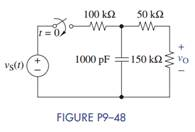### Create an Account

Home / Questions / The switch in Figure P9–48 has been open for a long time At t = 0 the switch is closed a ...

# The switch in Figure P9–48 has been open for a long time At t = 0 the switch is closed a Find the differential equation for the capacitor voltage and initial condition

The switch in Figure P9–48 has been open for a long time. At t = 0 the switch is closed.(a) Find the differential equation for the capacitor voltage and initial condition vC (0).

(b) Find vO(t) using the Laplace transformation for vS(t) = 100 u (t) V.

Jun 15 2020 View more View LessSubscribe To Get Solution